# ML之NB：利用NB朴素贝叶斯算法(CountVectorizer/TfidfVectorizer+去除停用词)进行分类预测、评估

+关注继续查看

## 输出结果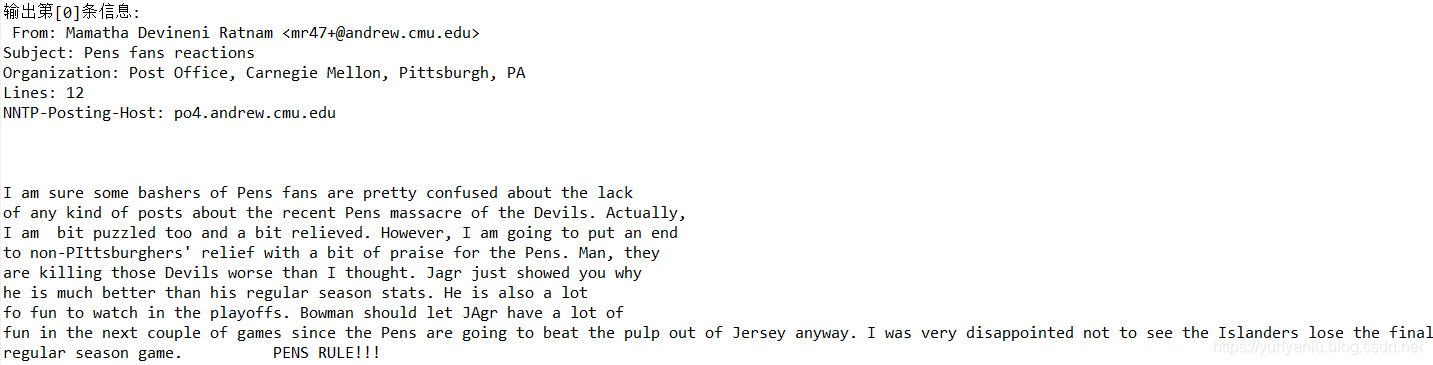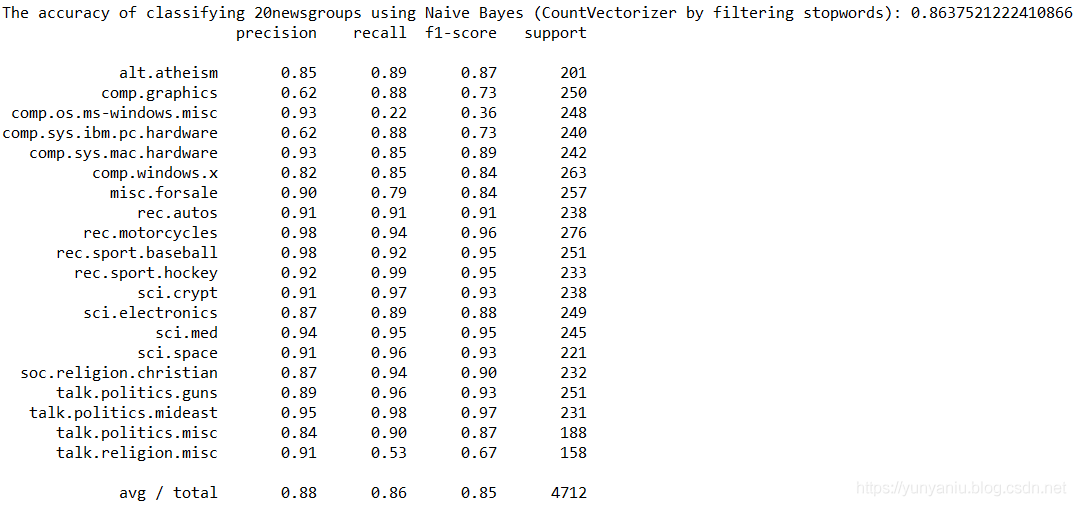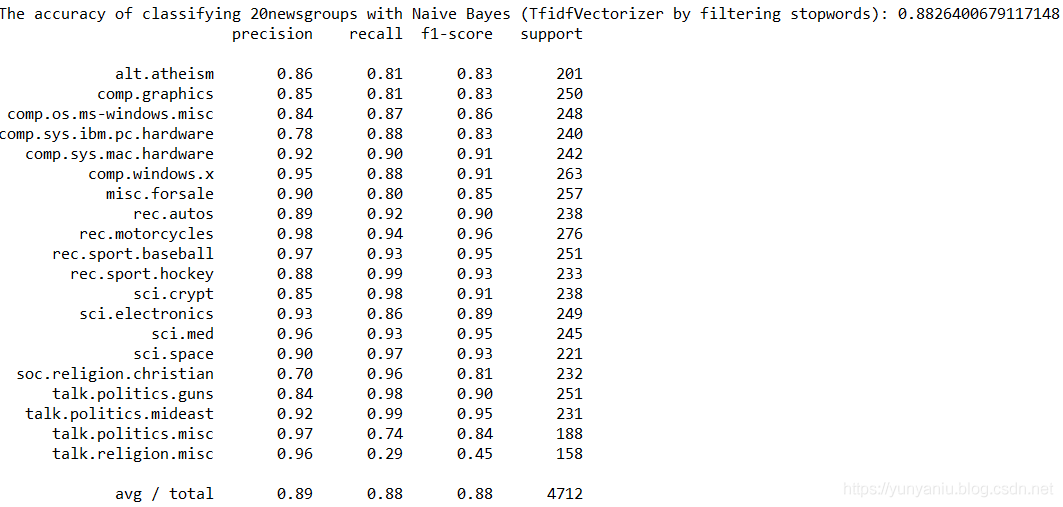## 设计思路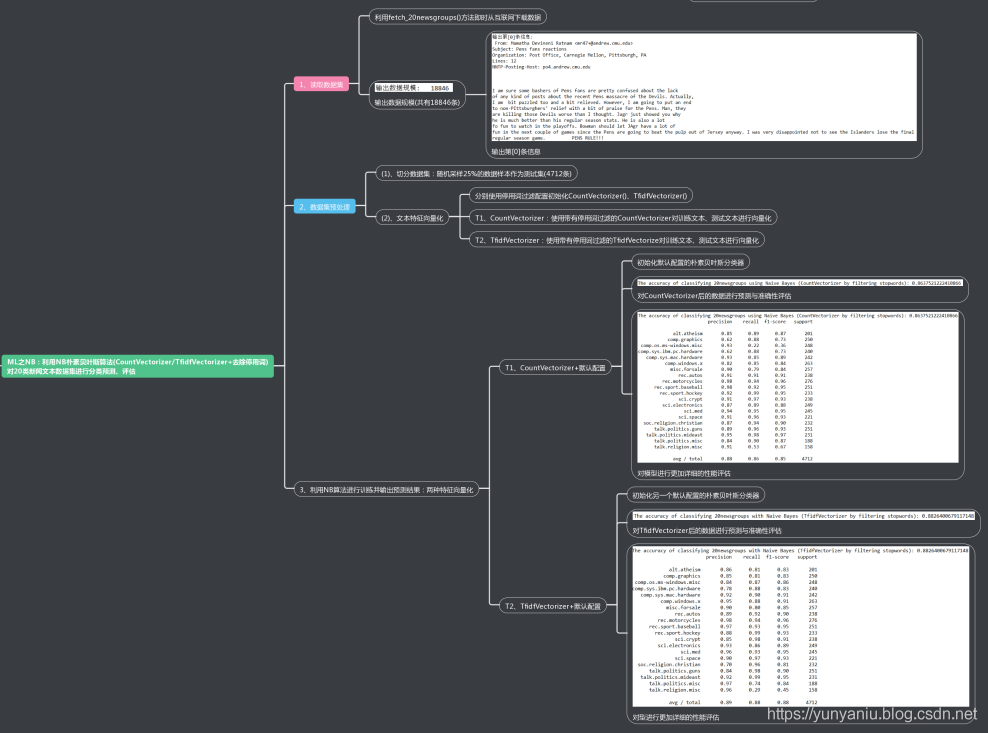## 核心代码

class CountVectorizer Found at: sklearn.feature_extraction.text

class CountVectorizer(BaseEstimator, VectorizerMixin):

"""Convert a collection of text documents to a matrix of token counts

This implementation produces a sparse representation of the counts using

scipy.sparse.csr_matrix.

If you do not provide an a-priori dictionary and you do not use an analyzer

that does some kind of feature selection then the number of features will

be equal to the vocabulary size found by analyzing the data.

Read more in the :ref:User Guide <text_feature_extraction>.

Parameters

----------

input : string {'filename', 'file', 'content'}

If 'filename', the sequence passed as an argument to fit is

expected to be a list of filenames that need reading to fetch

the raw content to analyze.

If 'file', the sequence items must have a 'read' method (file-like

object) that is called to fetch the bytes in memory.

Otherwise the input is expected to be the sequence strings or

bytes items are expected to be analyzed directly.

encoding : string, 'utf-8' by default.

If bytes or files are given to analyze, this encoding is used to

decode.

decode_error : {'strict', 'ignore', 'replace'}

Instruction on what to do if a byte sequence is given to analyze that

contains characters not of the given encoding. By default, it is

'strict', meaning that a UnicodeDecodeError will be raised. Other

values are 'ignore' and 'replace'.

strip_accents : {'ascii', 'unicode', None}

Remove accents during the preprocessing step.

'ascii' is a fast method that only works on characters that have

an direct ASCII mapping.

'unicode' is a slightly slower method that works on any characters.

None (default) does nothing.

analyzer : string, {'word', 'char', 'char_wb'} or callable

Whether the feature should be made of word or character n-grams.

Option 'char_wb' creates character n-grams only from text inside

word boundaries; n-grams at the edges of words are padded with space.

If a callable is passed it is used to extract the sequence of features

out of the raw, unprocessed input.

preprocessor : callable or None (default)

Override the preprocessing (string transformation) stage while

preserving the tokenizing and n-grams generation steps.

tokenizer : callable or None (default)

Override the string tokenization step while preserving the

preprocessing and n-grams generation steps.

Only applies if analyzer == 'word'.

ngram_range : tuple (min_n, max_n)

The lower and upper boundary of the range of n-values for different

n-grams to be extracted. All values of n such that min_n <= n <= max_n

will be used.

stop_words : string {'english'}, list, or None (default)

If 'english', a built-in stop word list for English is used.

If a list, that list is assumed to contain stop words, all of which

will be removed from the resulting tokens.

Only applies if analyzer == 'word'.

If None, no stop words will be used. max_df can be set to a value

in the range [0.7, 1.0) to automatically detect and filter stop

words based on intra corpus document frequency of terms.

lowercase : boolean, True by default

Convert all characters to lowercase before tokenizing.

token_pattern : string

Regular expression denoting what constitutes a "token", only used

if analyzer == 'word'. The default regexp select tokens of 2

or more alphanumeric characters (punctuation is completely ignored

and always treated as a token separator).

max_df : float in range [0.0, 1.0] or int, default=1.0

When building the vocabulary ignore terms that have a document

frequency strictly higher than the given threshold (corpus-specific

stop words).

If float, the parameter represents a proportion of documents, integer

absolute counts.

This parameter is ignored if vocabulary is not None.

min_df : float in range [0.0, 1.0] or int, default=1

When building the vocabulary ignore terms that have a document

frequency strictly lower than the given threshold. This value is also

called cut-off in the literature.

If float, the parameter represents a proportion of documents, integer

absolute counts.

This parameter is ignored if vocabulary is not None.

max_features : int or None, default=None

If not None, build a vocabulary that only consider the top

max_features ordered by term frequency across the corpus.

This parameter is ignored if vocabulary is not None.

vocabulary : Mapping or iterable, optional

Either a Mapping (e.g., a dict) where keys are terms and values are

indices in the feature matrix, or an iterable over terms. If not

given, a vocabulary is determined from the input documents. Indices

in the mapping should not be repeated and should not have any gap

between 0 and the largest index.

binary : boolean, default=False

If True, all non zero counts are set to 1. This is useful for discrete

probabilistic models that model binary events rather than integer

counts.

dtype : type, optional

Type of the matrix returned by fit_transform() or transform().

Attributes

----------

vocabulary_ : dict

A mapping of terms to feature indices.

stop_words_ : set

Terms that were ignored because they either:

- occurred in too many documents (max_df)

- occurred in too few documents (min_df)

- were cut off by feature selection (max_features).

This is only available if no vocabulary was given.

--------

HashingVectorizer, TfidfVectorizer

Notes

-----

The stop_words_ attribute can get large and increase the model size

when pickling. This attribute is provided only for introspection and can

be safely removed using delattr or set to None before pickling.

"""

def __init__(self, input='content', encoding='utf-8',

decode_error='strict', strip_accents=None,

lowercase=True, preprocessor=None, tokenizer=None,

stop_words=None, token_pattern=r"(?u)\b\w\w+\b",

ngram_range=(1, 1), analyzer='word',

max_df=1.0, min_df=1, max_features=None,

vocabulary=None, binary=False, dtype=np.int64):

self.input = input

self.encoding = encoding

self.decode_error = decode_error

self.strip_accents = strip_accents

self.preprocessor = preprocessor

self.tokenizer = tokenizer

self.analyzer = analyzer

self.lowercase = lowercase

self.token_pattern = token_pattern

self.stop_words = stop_words

self.max_df = max_df

self.min_df = min_df

if max_df < 0 or min_df < 0:

raise ValueError("negative value for max_df or min_df")

self.max_features = max_features

if max_features is not None:

if (not isinstance(max_features, numbers.Integral) or

max_features <= 0):

raise ValueError(

"max_features=%r, neither a positive integer nor None" %

max_features)

self.ngram_range = ngram_range

self.vocabulary = vocabulary

self.binary = binary

self.dtype = dtype

def _sort_features(self, X, vocabulary):

"""Sort features by name

Returns a reordered matrix and modifies the vocabulary in place

"""

sorted_features = sorted(six.iteritems(vocabulary))

map_index = np.empty(len(sorted_features), dtype=np.int32)

for new_val, (term, old_val) in enumerate(sorted_features):

vocabulary[term] = new_val

map_index[old_val] = new_val

X.indices = map_index.take(X.indices, mode='clip')

return X

def _limit_features(self, X, vocabulary, high=None, low=None,

limit=None):

"""Remove too rare or too common features.

Prune features that are non zero in more samples than high or less

documents than low, modifying the vocabulary, and restricting it to

at most the limit most frequent.

This does not prune samples with zero features.

"""

if high is None and low is None and limit is None:

return X, set()

# Calculate a mask based on document frequencies

dfs = _document_frequency(X)

tfs = np.asarray(X.sum(axis=0)).ravel()

if high is not None:

if low is not None:

if limit is not None and mask.sum() > limit:

new_indices = np.cumsum(mask) - 1 # maps old indices to new

removed_terms = set()

for term, old_index in list(six.iteritems(vocabulary)):

vocabulary[term] = new_indices[old_index]

else:

del vocabulary[term]

if len(kept_indices) == 0:

raise ValueError("After pruning, no terms remain. Try a lower"

" min_df or a higher max_df.")

:kept_indices], removed_terms

return X[

def _count_vocab(self, raw_documents, fixed_vocab):

"""Create sparse feature matrix, and vocabulary where

fixed_vocab=False

"""

if fixed_vocab:

vocabulary = self.vocabulary_

else:

# Add a new value when a new vocabulary item is seen

vocabulary = defaultdict()

vocabulary.default_factory = vocabulary.__len__

analyze = self.build_analyzer()

j_indices = []

indptr = _make_int_array()

values = _make_int_array()

indptr.append(0)

for doc in raw_documents:

feature_counter = {}

for feature in analyze(doc):

try:

feature_idx = vocabulary[feature]

if feature_idx not in feature_counter:

feature_counter[feature_idx] = 1

else:

feature_counter[feature_idx] += 1

except KeyError:

# Ignore out-of-vocabulary items for fixed_vocab=True

continue

j_indices.extend(feature_counter.keys())

values.extend(feature_counter.values())

indptr.append(len(j_indices))

if not fixed_vocab:

# disable defaultdict behaviour

vocabulary = dict(vocabulary)

if not vocabulary:

raise ValueError("empty vocabulary; perhaps the documents only"

" contain stop words")

j_indices = np.asarray(j_indices, dtype=np.intc)

indptr = np.frombuffer(indptr, dtype=np.intc)

values = np.frombuffer(values, dtype=np.intc)

X = sp.csr_matrix((values, j_indices, indptr),

shape=(len(indptr) - 1, len(vocabulary)),

dtype=self.dtype)

X.sort_indices()

return vocabulary, X

def fit(self, raw_documents, y=None):

"""Learn a vocabulary dictionary of all tokens in the raw documents.

Parameters

----------

raw_documents : iterable

An iterable which yields either str, unicode or file objects.

Returns

-------

self

"""

self.fit_transform(raw_documents)

return self

def fit_transform(self, raw_documents, y=None):

"""Learn the vocabulary dictionary and return term-document matrix.

This is equivalent to fit followed by transform, but more efficiently

implemented.

Parameters

----------

raw_documents : iterable

An iterable which yields either str, unicode or file objects.

Returns

-------

X : array, [n_samples, n_features]

Document-term matrix.

"""

# We intentionally don't call the transform method to make

# fit_transform overridable without unwanted side effects in

# TfidfVectorizer.

if isinstance(raw_documents, six.string_types):

raise ValueError(

"Iterable over raw text documents expected, "

self._validate_vocabulary()

max_df = self.max_df

min_df = self.min_df

max_features = self.max_features

vocabulary, X = self._count_vocab(raw_documents,

self.fixed_vocabulary_)

if self.binary:

X.data.fill(1)

if not self.fixed_vocabulary_:

X = self._sort_features(X, vocabulary)

n_doc = X.shape

max_doc_count = max_df if isinstance(max_df, numbers.Integral) else

max_df * n_doc

min_doc_count = min_df if isinstance(min_df, numbers.Integral) else

min_df * n_doc

if max_doc_count < min_doc_count:

raise ValueError(

"max_df corresponds to < documents than min_df")

X, self.stop_words_ = self._limit_features(X, vocabulary,

max_doc_count,

min_doc_count,

max_features)

self.vocabulary_ = vocabulary

return X

def transform(self, raw_documents):

"""Transform documents to document-term matrix.

Extract token counts out of raw text documents using the vocabulary

fitted with fit or the one provided to the constructor.

Parameters

----------

raw_documents : iterable

An iterable which yields either str, unicode or file objects.

Returns

-------

X : sparse matrix, [n_samples, n_features]

Document-term matrix.

"""

if isinstance(raw_documents, six.string_types):

raise ValueError(

"Iterable over raw text documents expected, "

if not hasattr(self, 'vocabulary_'):

self._validate_vocabulary()

self._check_vocabulary()

# use the same matrix-building strategy as fit_transform

_, X = self._count_vocab(raw_documents, fixed_vocab=True)

if self.binary:

X.data.fill(1)

return X

def inverse_transform(self, X):

"""Return terms per document with nonzero entries in X.

Parameters

----------

X : {array, sparse matrix}, shape = [n_samples, n_features]

Returns

-------

X_inv : list of arrays, len = n_samples

List of arrays of terms.

"""

self._check_vocabulary()

if sp.issparse(X):

# We need CSR format for fast row manipulations.

X = X.tocsr()

else:

# We need to convert X to a matrix, so that the indexing

# returns 2D objects

X = np.asmatrix(X)

n_samples = X.shape

terms = np.array(list(self.vocabulary_.keys()))

indices = np.array(list(self.vocabulary_.values()))

inverse_vocabulary = terms[np.argsort(indices)]

return [inverse_vocabulary[X[i:].nonzero()].ravel() for

i in range(n_samples)]

def get_feature_names(self):

"""Array mapping from feature integer indices to feature name"""

self._check_vocabulary()

return [t for t, i in sorted(six.iteritems(self.vocabulary_),

key=itemgetter(1))]28410 018979 0ML之Cosin：基于输入图片RGB均值化转为单向vector利用Cosin(余弦相似度)算法进行判别
ML之Cosin：基于输入图片RGB均值化转为单向vector利用Cosin(余弦相似度)算法进行判别
58 0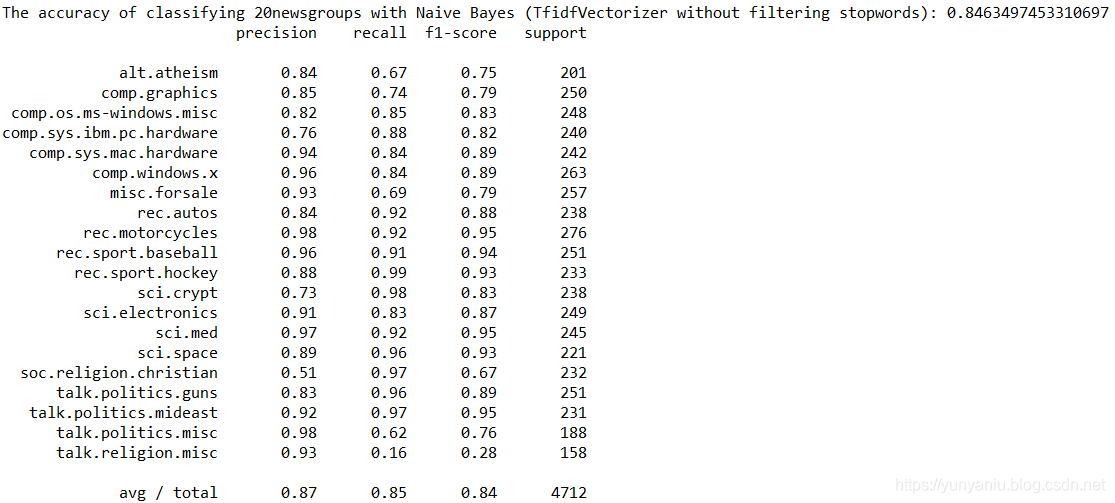ML之NB：利用朴素贝叶斯NB算法(TfidfVectorizer+不去除停用词)对20类新闻文本数据集进行分类预测、评估
ML之NB：利用朴素贝叶斯NB算法(TfidfVectorizer+不去除停用词)对20类新闻文本数据集进行分类预测、评估
80 0【算法与数据结构】一道检测inversion count的初级算法
（转载请注明出处：http://blog.csdn.net/buptgshengod） 1.题目            这是一道检测inversion count的算法。它将检测输入序列中反序输入的个数,即检测其中有几对A[i] &gt; A[j], i &lt; j 比如输入4，3，2，1，输出应该为3+2+1=6.。 因为： 1.  4比3，2，1大，但4在输入序列
755 0CMU风头被抢，新型DeepStack算法抢先攻克德州扑克图灵测试
1542 0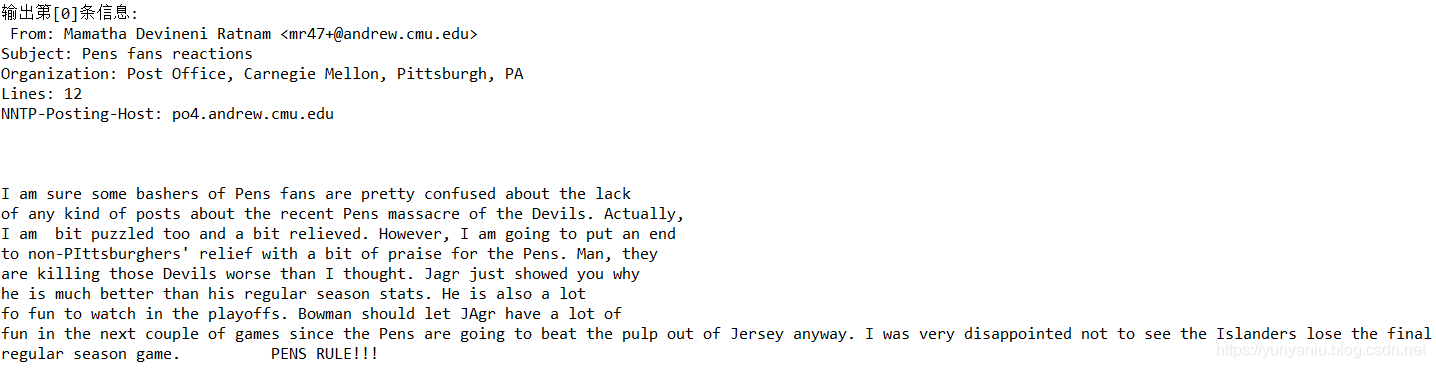ML之NB：利用朴素贝叶斯NB算法(CountVectorizer+不去除停用词)对fetch_20newsgroups数据集(20类新闻文本)进行分类预测、评估
ML之NB：利用朴素贝叶斯NB算法(CountVectorizer+不去除停用词)对fetch_20newsgroups数据集(20类新闻文本)进行分类预测、评估
84 0ML之NB：利用NB朴素贝叶斯算法(CountVectorizer/TfidfVectorizer+去除停用词)进行分类预测、评估
ML之NB：利用NB朴素贝叶斯算法(CountVectorizer/TfidfVectorizer+去除停用词)进行分类预测、评估
82 020325 0Facebook 开源 Zstandard 算法及 MyRocks 存储引擎
1555 0

1702

0

JS零基础入门教程（上册）